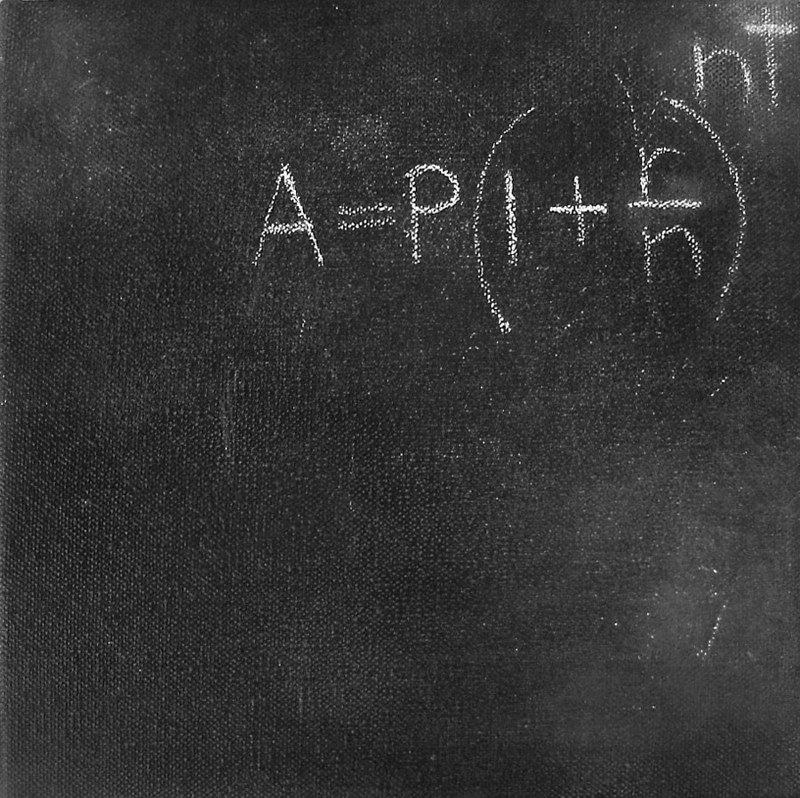Writing with Light, How to Become a Millionairess (Secret #1)

mixed media on cotton canvas 20 x 20cm 2011
[The work is based on the formula for calculating compound interest, where A = final amount, P = principal amount (initial investment), r = annual nominal interest rate (as a decimal), n = number of times the interest is compounded per year, and t = number of years).]